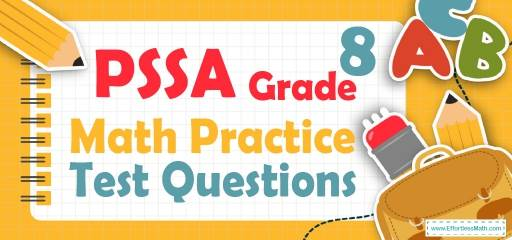# 8th Grade PSSA Math Practice Test QuestionsPreparing your student for the 8th Grade PSSA Math test? Help your students build PSSA Math test skills by following common PSSA Math questions for 8th Grade. Practicing common math questions is the best way to help your students improve their Math skills and prepare for the test. Here, we provide a step-by-step guide to solve 10 common PSSA Math practice problems covering the most important math concepts on the 8th Grade PSSA Math test.

## 10 Sample 8th Grade PSSA Math Practice Questions

1- Five years ago, Amy was three times as old as Mike was. If Mike is 10 years old now, how old is Amy?

A. 4

B. 8

C. 12

D. 20

2- What is the length of AB in the following figure if $$AE=4, CD=6$$ and $$AC=12$$?

A. 3.8

B. 4.8

C. 7.2

D. 24

3- If a gas tank can hold 25 gallons, how many gallons does it contain when it is $$\frac{2}{5}$$ full?

A. 50

B. 125

C. 62.5

D. 10

4- In the xy-plane, the point $$(4,3)$$ and $$(3,2)$$ are on line $$A$$. Which of the following equations of lines is parallel to line $$A$$?

A. $$y=3x$$

B. $$y=\frac{x}{2}$$

C. $$y=2x$$

D. $$y=x$$

5- If $$x$$ is directly proportional to the square of $$y$$, and $$y=2$$ when $$x=12$$, then when $$x=75 y=$$ ?

A. $$\frac{1}{5}$$

B. 1

C. 5

D. 12

6- Jack earns $616 for his first 44 hours of work in a week and is then paid 1.5 times his regular hourly rate for any additional hours. This week, Jack needs$826 to pay his rent, bills, and other expenses. How many hours must he work to make enough money in this week?

A. 40

B. 48

C. 53

D. 54

7-

If a is the mean (average) of the number of cities in each pollution type category, b is the mode, and c is the median of the number of cities in each pollution type category, then which of the following must be true?

A. $$a<b<c$$

B. $$b<a<c$$

C. $$a=c$$

D. $$b<c=a$$

8-

What percent of cities are in the type of pollution $$A, C,$$ and $$D$$ respectively?

A. $$60\%, 40\%, 90\%$$

B. $$30\%, 40\%, 90\%$$

C. $$30\%, 40\%, 60\%$$

D. $$40\%, 60\%, 90\%$$

9-

How many cities should be added to the type of pollution $$B$$ until the ratio of cities in the type of pollution $$B$$ to cities in the type of pollution $$E$$ will be 0.625?

A. 2

B. 3

C. 4

D. 5

10- In the following right triangle, if the sides $$AB$$ and $$AC$$ become twice longer, what will be the ratio of the perimeter of the triangle to its area?

A. $$\frac{1}{2}$$

B. 2

C. $$\frac{1}{3}$$

D. 3

## Best 8th Grade PSSA Math Prep Resource for 2022

1- D
Five years ago, Amy was three times as old as Mike. Mike is 10 years now. Therefore, 5 years ago Mike was 5 years.
Five years ago, Amy was: $$A=3×5=15$$
Now Amy is 20 years old: $$15 + 5 = 20$$

2- B
Two triangles $$∆BAE$$ and $$∆BCD$$ are similar. Then:
$$\frac{AE}{CD}=\frac{AB}{BC}=\frac{4}{6}=\frac{x}{12}$$
$$→48-4x=6x→10x=48→x=4.8$$

3- D
$$\frac{2}{5}×25=\frac{50}{5}=10$$

4- D
The slop of line A is:
$$m = \frac{y_2-y_1}{x_2-x_1}=\frac{3-2}{4-3}=1$$
Parallel lines have the same slope and only choice $$D (y=x)$$ has slope of 1.

5- C
$$x$$ is directly proportional to the square of $$y$$. Then:
$$x=cy^2$$
$$12=c(2)^2→12=4c→c=\frac{12}{4}=3$$
The relationship between $$x$$ and $$y$$ is:
$$x=3y^2$$
$$x=75$$
$$75=3y^2→y^2=\frac{75}{3}=25→y=5$$

6- D
he amount of money that jack earns for one hour: $$\frac{616}{44}=14$$
Number of additional hours that he work to make enough money is: $$\frac{826-616}{1.5×14}=10$$
Number of total hours is: $$44+10=54$$

7- C
Let’s find the mean (average), mode and median of the number of cities for each type of pollution.
Number of cities for each type of pollution: $$6, 3, 4, 9, 8$$
$$mean (average) = \frac{sum \space of \space terms}{number \space of \space terms}=\frac{6+3+4+9+8}{5}=6$$
Median is the number in the middle. To find median, first list numbers in order from smallest to largest.
$$3, 4, 6, 8, 9$$
Median of the data is 6.
Mode is the number that appears most often in a set of numbers. Therefore, there is no mode in the set of numbers.
$$Median = Mean, then, a=b$$

8- A
Percent of cities in the type of pollution A: $$\frac{6}{10} × 100=60\%$$
Percent of cities in the type of pollution C: $$\frac{4}{10} × 100 = 40\%$$
Percent of cities in the type of pollution D: $$\frac{9}{10}× 100 = 90\%$$

9- A
Let the number of cities should be added to type of pollutions $$B$$ be $$x$$. Then:
$$\frac{x + 3}{8}=0.625→x+3=8×0.625→x+3=5→x=2$$

10- A
$$AB=12$$ And $$AC=5$$
$$BC=\sqrt{(12^2+5^2 )} = \sqrt{(144+25)} = \sqrt{169}=13$$
$$Perimeter =5+12+13=30$$
$$Area =\frac{5×12}{2}=5×6=30$$
In this case, the ratio of the perimeter of the triangle to its area is:
$$\frac{30}{30}= 1$$
If the sides $$AB$$ and $$AC$$ become twice longer, then:
$$AB=24$$ And $$AC=10$$
$$BC=\sqrt{(24^2+10^2 )} = \sqrt{(576+100)} = \sqrt{676} = 26$$
Perimeter $$=26+24+10=60$$
$$Area =\frac{10×24}{2}=10×12=120$$
In this case the ratio of the perimeter of the triangle to its area is:
$$\frac{60}{120}=\frac{1}{2}$$

Looking for the best resource to help you succeed on the PSSA Math Grade 8 Math test?

## The Best Books to Ace 8th Grade PSSA Math Test

### What people say about "8th Grade PSSA Math Practice Test Questions - Effortless Math: We Help Students Learn to LOVE Mathematics"?

No one replied yet.

X
30% OFF

Limited time only!

Save Over 30%

SAVE $5 It was$16.99 now it is \$11.99